# Preparation of Zinc Oxide Nanomaterial and Research and Development of Antibacterial Property

Preparation of Zinc Oxide Nanomaterial and Research and Development of Antibacterial Property
School of Environment Design, Hunan International Economics University, Changsha 410205, China

Corresponding Author Email:
huangjia1204@163.com
Page:
407-415
|
DOI:
https://doi.org/10.18280/acsm.450507
13 July 2021
|
Accepted:
20 August 2021
|
Published:
31 October 2021
| Citation

OPEN ACCESS

Abstract:

Children using environmentally unfriendly furniture are more likely to suffer from allergic rhinitis, respiratory infections, and leukemia. The green design of children’s furniture must consider anti-formaldehyde, deodorant, antibacterial, and antiviral factors. This paper prepares a zinc oxide (ZnO) nanomaterial for children’s furniture, and researches and develops the antibacterial property of the nanomaterial. The main contents include the synthesis method and preparation process, material morphology and structure characterization, and antibacterial performance testing. The practical substrate modification of the material was realized by pre-depositing polydopamine particles. In this way, the ZnO nanomaterial could apply to a wider range of children’s furniture, become more durable, and realize efficient multi-mechanism synergistic sterilization. Experimental results show that the prepared material has a good antibacterial property.

Keywords:

zinc oxide (ZnO) nanomaterial, preparation, antibacterial property, children’s furniture

1. Introduction

Green living is an increasingly popular concept. Safe and high-quality green furniture, especially children’s wooden furniture, has become the core demand of consumers [1-6]. Children using environmentally unfriendly furniture are more likely to suffer from allergic rhinitis, respiratory infections, and leukemia [7-12]. The green design of children’s furniture must consider all the factors that might endanger their health, such as anti-formaldehyde, deodorant, antibacterial, and antiviral factors [13-17]. Zinc oxide (ZnO) nanomaterial provides a special paint for children’s furniture, enabling functions like sterilization, health care, formaldehyde removal, stain resistance, and anti-aging.

Hermida-Montero et al.  reported the in vitro antifungal activity of ZnO nanoparticles against three symbiotic fungi of ragweed beetle, and studied the coating and particle size of two types of ZnO nanoparticles on antifungal activity. Experiments show that, although nanoparticle size is a key parameter, the coating of ZnO nanoparticles is the leading contributor to antifungal performance. La et al.  prepared ZnO nanoparticles by hydrothermal method, and characterized them by scanning electron microscopy (SEM) and X-ray diffraction (XRD). The prepared ZnO nanoparticles showed good antibacterial properties against several bacteria, including Staphylococcus aureus, Escherichia coli and Bacillus subtilis. Li et al.  presented a simple method for preparing superhydrophobic coatings based on acrylic resins: ZnO nanoparticles are modified with silane to obtain hydrophobic ZnO, which is uniformly blended with acrylic resin, and then the mixture is sprayed onto the aluminum plate, forming a cured coating. Experimental results verify that the antibacterial performance of the ZnO nanoparticles/acrylic resin superhydrophobic coating is much better than pure acrylic resin coating.

The hydrophobicity and color stability are important features of wood. When wood is used as a raw material for outdoor products, these features are easy to change, thereby shortening the service life of the wood. Tuong et al.  adopted a two-step simple spraying method to apply ZnO coating to benzoin wood, aiming to improve hydrophobicity and color stability of the wood, the hydrophobicity and firmness of the coating, and the color stability of uncoated wood samples. Then, they evaluated the coated wood samples. Pang et al.  prepared A ZnO/TiO2 nano-array coating through hydrothermal synthesis and low-temperature liquid phase method. In vitro antibacterial experiments show that the bacterium has a maximum inhibitory rate of 99% against Staphylococcus aureus and 90% against Escherichia coli.

This paper focuses on the preparation of a ZnO nanomaterial for children's furniture, and the research and development of antibacterial property. The main contents are as follows: After summing up the strengths and defects of ZnO nanomaterial, Section 2 specifies the principle and flow of preparing Zinc nano-array for high-temperature hard materials like metal handles, and wooden plates in children’s furniture, respectively, and analyzes the antibacterial mechanism and antibacterial performance of the zinc nano-array. Section 3 improves the enrichment of ZnO nanocrystals on cellulose by pre-depositing polydopamine particles, and explains the process of antibacterial performance determination and thermogravimetric analysis. Section 4 carries out comparative experiments to demonstrate the good antibacterial property of the prepared modified material.

2. Preparation and Antibacterial Property of ZnO Nano-Array

2.1 Preparation and characterization

Whether the environment is bright or dark, the ZnO, as an active oxide produced by catalytic reaction, always has an excellent antibacterial effect. However, the chemical antibacterial mechanism depends on the release of active oxygen, and generally faces problems like the slow active effect, the short life of active oxides, the potential microbial mutation, and the development of drug resistance. To solve these problems, it is particularly important to find a new antibacterial method. Inspired by the physical sterilization of bionic nanostructures, some scholars have constructed ZnO nano-arrays, which not only pierce through bacteria, but also achieve significant photocatalytic self-cleaning and functional regeneration.

Taking nano-ZnO array as the object, this paper describes the synthesis method and the preparation process of nano-ZnO array, characterizes the morphology and structure of the material, and changes and modifies the practical substrate. In this way, the ZnO nanomaterial could apply to a wider range of children’s furniture, become more durable, and realize efficient multi-mechanism synergistic sterilization. Tables 1 and 2 list the reagents and instruments/equipment of our experiments.

ZnO has multiple crystal structures. The hydrothermal method of ZnO nano-array includes three primary stages: the preparation of the precursor solution of the seed layer, the preparation of the seed layer, and the generation of the nano-array. For high-temperature hard materials like the metal handles of children’s furniture, the pulling method can be adopted to generate a ZnO array on a clean and dry rigid substrate. During the generation, the required crystal ion Zn2+ is provided by zinc nitrate hexahydrate, and the required OH- is supplied by the hydrolysis of hexamethylenetetramine. The specific reactions are described by:

$6{{H}_{2}}O+{{\left( C{{H}_{2}} \right)}_{6}}{{N}_{4}}\to 4N{{H}_{3}}+6HCHO$                (1)

${{H}_{2}}O+N{{H}_{3}}\to O{{H}^{-}}+NH_{4}^{+}$                 (2)

$2O{{H}^{-}}+Z{{n}^{2+}}\to Zn{{\left( OH \right)}_{2}}$                   (3)

$2O{{H}^{-}}+Zn{{\left( OH \right)}_{2}}\to Zn\left( OH \right)_{4}^{2-}$                  (4)

$Zn\left( OH \right)_{4}^{2-}+Zn\left( OH \right)_{4}^{2-}\to 2{{H}_{2}}O+4{{\left( OH \right)}^{-}}+2ZnO\downarrow$                  (5)

During the reactions of the pulling method, the precursor solution of the seed layer forms an even layer of zinc nitrate hexahydrate liquid on high-temperature hard materials like stainless steel sheet, stainless steel mesh, copper sheet, and aluminum sheet. After four or more times of the above pulling and annealing, a seed layer can be obtained from the substrate. Then, the substrate plate is placed in the growth solution for a certain period, and then flushed, dried, and annealed. The reinforced crystalline samples of the residual organic matter can thereby be obtained. Figure 1 shows the flow of generating ZnO nano-array based on high-temperature hard materials.

The ZnO nano-array can also be obtained through hydrothermal operation on the surface of impregnated fibrous material, such as the wood-based boards of children’s furniture. Figure 2 explains the flow for generating ZnO nano-array based on fibrous materials. However, the active substrate of the cellulose, different from other substrates, can be complexed with zinc ions. The complexation strengthens the bond between ZnO and the substrate. The specific reactions are given as follows:

$Z{{n}^{2+}}+Cell\cdot {{O}^{-}}\to Cell\cdot O\cdot Z{{n}^{+}}$               (6)

$2O{{H}^{-}}+Cell\cdot O\cdot Z{{n}^{+}}\to Cell\cdot O\cdot Zn\left( OH \right)_{2}^{-}$                   (7)

$2O{{H}^{-}}+Cell\cdot O\cdot Zn\left( OH \right)_{2}^{-}\to Cell\cdot O\cdot Zn\left( OH \right)_{4}^{3-}$                   (8)

Table 1. Reagents

 Name Specification Manufacturer Dihydrate zinc nitrate Analytically pure Tianjin Guangfu (China) Sodium hydroxide Analytically pure Tianjin Fengchuan (China) Isopropanol Analytically pure Tianjin Yingcheng (China) Dihydrate ammonium acetate Analytically pure Tianjin Guangfu (China) Absolute ethyl alcohol Analytically pure Tianjin Yingcheng (China) Hexamethylenetetramine Analytically pure Tianjin Fengchuan (China) Polyethylene glycol octylphenyl ether Analytically pure Tianjin Fengchuan (China) Citric acid Analytically pure Tianjin Fengchuan (China) Triethylamine Analytically pure Tianjin Fengchuan (China) Bacterial cellulose Food grade Hainan Yide (China)

Table 2. Instruments and equipment

 Name Manufacturer Model Electronic balance Adam Equipment, Wuhan (China) PWC124 Thermal field emission scanning electron microscope OLimp (Japan) TF2000 High-temperature and high-pressure autoclave Jiangyin Binjiang (China) YK-24HDD Freeze dryer Ningbo Scientz (China) SCIENTZ-10N Electro-heating standing-temperature cultivator Shanghai Yuejin (China) HH.B-S Constant temperature water bath Gonyi Yuhua (China) CH1015 High-speed refrigerated centrifuge Eppendorf (Germany) 3S10R Water bath oscillator Haimen Kylin-Bell (China) QL-901 X-ray diffractometer Rigaku (Japan) D/MAX-2500 Cell incubator Thermal Fisher Scientific (United States) Therme371 Thermal gravimetric analyzer Netzsch (Germany) STA4499F3 Universal testing machine Instron (United States) Instron3369 Electric furnace Beijing Zhongxingweiye (China) DL-1

## 1.png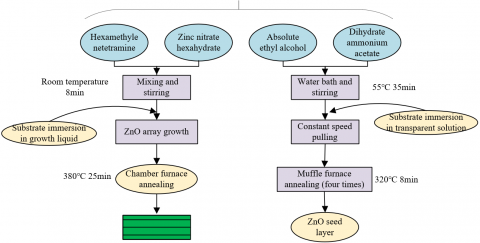Figure 1. Experimental flow of high-temperature hard materials

$Cell\cdot O\cdot Zn\left( OH \right)_{4}^{3-}\to {{H}_{2}}O+Cell\cdot O\cdot ZnO$                 (9)

$2O{{H}^{-}}+Z{{n}^{2+}}\to Zn{{\left( OH \right)}_{2}}$                    (10)

$2O{{H}^{-}}+Zn{{\left( OH \right)}^{2}}\to Zn\left( OH \right)_{4}^{2-}$                  (11)

$Zn\left( OH \right)_{4}^{2-}+Zn\left( OH \right)_{4}^{2-}\to 4{{\left( OH \right)}^{-}}+2{{H}_{2}}O+2ZnO\downarrow$                  (12)

The structure of the obtained ZnO crystals was characterized by an X-ray diffractometer at room temperature. Let MG be the mean grain size; ψ be the X-ray wavelength; α be half peak width; ω[3, 45°] be the Bragg diffraction angle. Then, the mean grain size of ZnO can be calculated by:

$MG=0.89\psi /\left( \alpha cos\omega \right)$                 (13)

Let QC be the number-average molecular weight; QH be the weight-average molecular weight. Tetrahydropyrane and polystyrene were selected as the solvent and the standard sample, respectively. Then, a gel permeation chromatographic analyzer was employed to measure the QC and QH of the obtained ZnO crystals. The polydispersity index can be calculated by:

## 2.png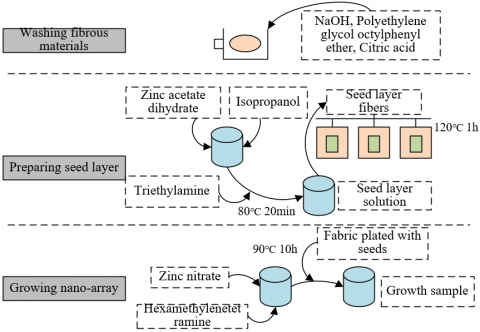Figure 2. Experimental flow of fibrous materials

$PDI=\frac{{{Q}_{H}}}{{{Q}_{C}}}$                     (14)

Let ε be the mean grain diameter; ψ1/2 be the wavelength at the excitation peak or the half-absorption position. Based on the empirical relationship between ε and ψ1/2 of ZnO, the particle size of ZnO crystals can be calculated by:

$\frac{1240}{{{\psi }_{1/2}}}=3.301+\frac{294.0}{{{\varepsilon }^{2}}}+\frac{1.09}{\varepsilon }$                   (15)

Let RQZnO be the recovery mass of ZnO nanocrystals. Then, the yield η of ZnO nanomaterials can be calculated by:

$\eta=\frac{R Q_{Z n O}}{R Q_{(CH_{2})_{6}} \quad N_{4}, 6 H_2 O \quad+R Q_{N H_{3}, H_{2} O} \quad +R Q_{O H^{-}} \quad+R Q_{Z n(O H)_{2}}}$                 (16)

2.2 Determination of antibacterial property

The active carboxyl content of cellulose was determined according to the standard TAPPI T237 cm-98. Firstly, a proper amount of cellulose was taken from flexible fibrous materials, treated in hydrochloric acid solution for 2h, and flushed with deionized water. Then, NaHCO3-NaCl solution was quickly added to the conical flash containing the filtrate. After 1h of reaction, Methyl red indicator was added to the filtrate. Finally, titration was carried out based on hydrochloric acid solution. Let E and F be the hydrochloric acid solution used for titrating the filtrate, and for titrating the NaHCO3-NaCl solution, respectively; G be the mass of water in the wet slurry cake obtained through the flushing by deionized water; P be the actual concentration of the hydrochloric acid solution; Z be the absolute dry mass of the cellulose sample. Then, the active carboxyl content of cellulose can be calculated by:

$CAS=\left\{ F-\left[ E+\left( E\times G/50 \right) \right] \right\}\times P\times \left( 200/Z \right)$                    (17)

The nitrogen content in and on the zinc acetate solution film were measured by an elemental analyzer. Since ZnO nanomaterials contain no nitrogen, the nitrogen must come from the chitosan of cellulose. Let W and D be the mass of the zinc acetate solution film before and after flushing, drying, and annealing, respectively. Then, the mass percentage of chitosan on the surface of zinc acetate solution film can be calculated by:

$CS{\scriptstyle{}^{0}/{}_{0}}=D/W\times 100{\scriptstyle{}^{0}/{}_{0}}$                   (18)

Next, Botrytis cinerea, Escherichia coli, and Staphylococcus aureus of cell density 1×106/mL were inoculated to the crystallized cellulose substrate. On the 1st and 3rd of curing, cell counting was performed with a Cell Counting Kit-8 (CCK-8). Let EGAV and NGAV be the absorbance value of the experimental group, and the absorbance value of the negative group, respectively. Then, the relative cell proliferation rate can be calculated by:

$RCPR=EGAV/NGAV\times 100{\scriptstyle{}^{0}/{}_{0}}$                    (19)

The diffusion and growth scope of different colonies on each substrate was measured by a vernier caliper. Let GRO be the mycelial growth range of the reference substrate, and GRF be that of the substrate containing the ZnO nano-array. Then, the bacteriostasis rate ηIR of ZnO nano-array on each colony can be calculated by:

${{\eta }_{IR}}=\frac{G{{R}_{O}}-G{{R}_{F}}}{G{{R}_{O}}}\times 100{\scriptstyle{}^{0}/{}_{0}}$                    (20)

3. Preparation and Antibacterial Properties of Modified ZnO Nanomaterials

3.1 Preparation and characterization

The dopamine polymer with functional group like primary amine and indole is strongly adhesive and universally applicable. It can deposit on polymers, hard materials, and flexible fibrous materials. If a flexible fibrous material is pre-deposited with polydopamine, the ZnO nanocrystals will concentrate on the cellulose more apparently.

## 3.png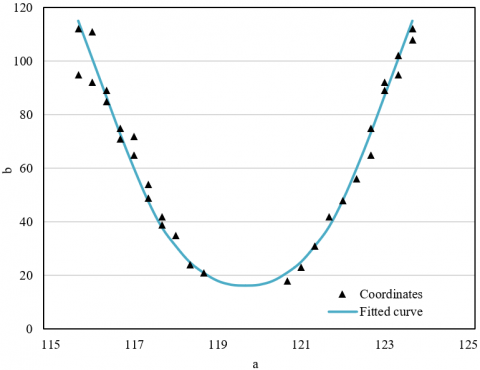Figure 3. The curve fitted by GaussAMP function

To further determine the relative content of ZnO nanocrystals produced before and after the pre-deposition of polydopamine particles, this paper carries out a mathematical statistical analysis on ZnO nanocrystals based on image processing techniques. Firstly, Photoshop was adopted to uniformly take several pixels from the ZnO nano-array. Then, the coordinates of each pixel were imported to the function plotting tool Origin 8.5 to draw scatterplots. Finally, each pixel was fitted. The GaussAMP function is employed here:

$b={{b}_{0}}+\delta {{e}^{-\frac{{{\left( a-{{a}_{v}} \right)}^{\quad 2}}}{2{{q}^{2}}}}}$                  (21)

The curve fitted by GaussAMP function is shown in Figure 3, where a(-2.2,2.2); b0, δ, and 2q2 equal -274562, 336452, and 57425, respectively.

Under room temperature, the difference between the two crystal faces before and after the pre-deposition of polydopamine particles can be obtained using ZnO nanocrystal parameters. The difference was characterized by the idea of calculus. Let T and T’ be the number of ZnO nanocrystals before and after the pre-deposition of polydopamine particles, respectively; u and e be the total length and width of the exposed crystal surface, respectively. Then, the ratio of the exposed crystals before and after the pre-deposition of polydopamine particles can be calculated by:

$S=\frac{\sum{{{T}^{2}}ue}}{\sum{{{{{T}'}}^{2}}ue}}$              (22)

3.2 Determination of antibacterial property

Concerning the antibacterial activity of nano-zinc oxide materials in darkness, this paper only considers the final products H2O2 and $O_{2}^{-}$.

Following the KI oxidation-reduction titration, the H2O2 produced from the ZnO suspension was determined by measuring the absorbance with a visible spectrophotometer. The measurement was conducted in a completely dark environment. Let XG450 be the absorbance value at 450 nm; v be the H2O2 content of the solution. Then, the linear regression equation for the standard curve between the H2O2 content and absorbance can be fitted as:

$X{{G}_{450}}=0.132v-0.0065$                (23)

Let Vu and Vq be the H2O2 contents in u bottles and q bottles of sample solution, respectively; SO be the sampling volume. Then, the yield of the active substance H2O2 in the suspension can be calculated by:

$v=\frac{9\left( {{V}_{U}}-{{V}_{Q}} \right)\left( X{{G}_{450}} \quad+0.0065 \right)}{0.6\times 0.132\times SO}$                  (24)

The $O_{2}^{-}$ content was estimated by nitro blue tetrazolium. Let XG290 be the absorbance value at 290 nm; v' be the $O_{2}^{-}$ content in the solution. Then, the linear regression equation for the standard curve between the nitro blue tetrazolium content and absorbance can be fitted as:

$X{{G}_{290}}=0.0421{v}'+0.01448$                  (25)

Let Vu’ and Vq’ be the $O_{2}^{-}$ contents in u bottles and q bottles of sample solution, respectively; SO’ be the sampling volume. Then, the yield of the active substance $O_{2}^{-}$ in the suspension can be calculated by:

${v}'=\frac{9\left( {{{{V}'}}_{U}}-{{{{V}'}}_{Q}} \right)\left( X{{G}_{290}}\quad+0.0077 \right)}{0.45\times 0.174\times S{O}'}$                    (26)

Finally, the antibacterial activity of the prepare ZnO nanoparticles was verified in a dark environment, using three common type strains of the cell density 1×106/mL: Botrytis cinerea, Escherichia coli, and Staphylococcus aureus.

3.3 Thermogravimetric analysis

Let φCR and φAP be the diffraction peak area of the crystalline phase and the amorphous phase, respectively. The crystallinity of ZnO nanocrystals generated before and after the pre-deposition of polydopamine particles can be calculated by

$CRY={{\phi }_{CR}}/\left( {{\phi }_{CR}}+{{\phi }_{AP}} \right)\times 100{\scriptstyle{}^{0}/{}_{0}}$                 (27)

For the modified antibacterial material based on the prepared ZnO nanocrystals, the mechanical properties can be characterized by breaking strength. Let RI, BS, HD, and KD be the relative strength, breaking strength, thickness, and width of the material, respectively. Then, we have:

$RI=\frac{BS}{HD\times KD}$                     (28)

To further explore the influence of nanoparticle properties over the thermal degradation of modified ZnO nanomaterials, this paper analyzes the modified antibacterial materials based on the thermal degradation reaction kinetics of modified materials. Let POs, POτ, and POe be the weights of the modified material at the start, at time τ, and at the end of the thermal degradation reaction. Then, the weight loss rate of the modified material can be calculated by:

$\gamma =\frac{P{{O}_{s}}-P{{O}_{\tau }}}{P{{O}_{s}}-P{{O}_{e}}}$                  (29)

The thermal degradation rate can be calculated by:

$\frac{d\gamma }{d\tau }=\Psi g\left( \gamma \right)$                   (30)

According to the empirical formula of the relationship between chemical reaction rate and temperature, we have:

$\Psi =SH\cdot {{e}^{-Q{{B}_{c}}/MG\xi }}$                 (31)

where, Ψ is the rate constant; MG is molar gas constant, ξ is thermodynamic temperature; QBC is apparent activation energy; SH is pre-exponential factor. Let m be the number of reaction stages. The reaction mechanism of the modified material directly determines the function g (γ) in formula (30):

$g\left( \gamma \right)={{\left( 1-\gamma \right)}^{m}}$                  (32)

Combining formulas (30)-(32):

$\frac{d\gamma }{d\tau }=SH\cdot {{e}^{-\frac{Q{{B}_{c}}}{MG\xi }}}{{ \quad \left(1-\gamma \right)}^{m}}$                    (33)

Taking the log of both sides of formula (33) and finding differentials of dγ/dτ, and 1-γ:

$\Delta ln\left( \frac{d\gamma }{d\tau } \right)=-\frac{Q{{B}_{c}}}{3.15S}\cdot d\left( 1/\xi \right)+mdln\left( 1-\gamma \right)$                  (34)

Formula (34) can be converted to a differential form:

$\Delta ln\left( \frac{d\gamma }{d\tau } \right)=-\frac{Q{{B}_{c}}}{3.15S}\cdot \Delta \left( 1/\xi \right)+m\Delta ln\left( 1-\gamma \right)$               (35)

Dividing both sides of formula (35) by ln(1-γ):

$\frac{\Delta ln\left( d\gamma /d\tau \right)}{\Delta ln\left( 1-\gamma \right)}=-\frac{Q{{B}_{c}}}{3.15S}\cdot \frac{\Delta \left( 1/\xi \right)}{\Delta ln\left( 1-\gamma \right)}+m$                  (36)

Since dγ/dτ=(dγ/dξ)×(dξ/dτ), we have:

$\frac{\Delta ln\left( d\gamma /d\tau \right)}{\Delta ln\left( 1-\gamma \right)}=\frac{\Delta ln\left( d\gamma /d\xi \right)}{\Delta ln\left( 1-\gamma \right)}$                        (37)

That is:

$\frac{\Delta ln\left( d\gamma /d\xi \right)}{\Delta ln\left( 1-\gamma \right)}=-\frac{Q{{B}_{c}}}{3.15S}\cdot \frac{\Delta \left( 1/\xi \right)}{\Delta ln\left( 1-\gamma \right)}+m$                    (38)

4. Experiments and Results Analysis

## 4a.png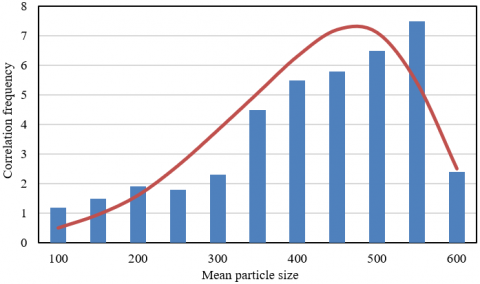(a)

## 4b.png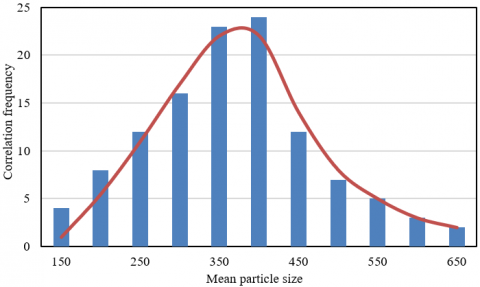(b)

## 4c.png(c)

## 4d.png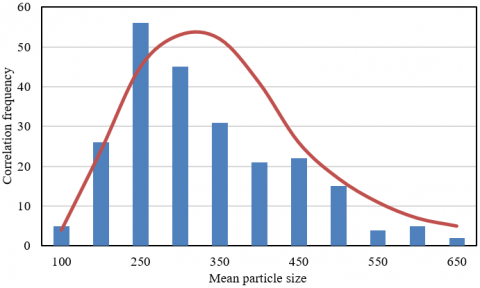(d)

Figure 4. Particle size distribution of ZnO nanocrystals

Figure 4 shows the particle size distribution of the modified ZnO nanocrystals obtained with growth solution of different concentrations: 6mmol/L, 12mmol/L, 24mmol/L, and 30mmol/L. It can be seen that growth solution concentration significantly affected the particle size distribution of the ZnO crystals in the modified material. As the growth solution concentration increased from 6mmol/L to 30mmol/L, the mean particle size of ZnO nanocrystals first increased and then gradually decreased. This means the rising concentration of the growth solution pushes up the zinc ion content, and the number of ZnO crystals. As a result, more active sites appear as zinc ions are absorbed by the cellulose substrate. When the growth solution reaches a certain concentration, the zinc ions outside the cellulose substrate are directly bonded, impeding the crystallization of ZnO on substrate surface. Hence, the number and mean particle size of ZnO crystals both dropped gradually.

Table 3 provides the statistics on the particle size and antibacterial property of ZnO nanocrystals with growth solution of different concentrations. The largest quantity and most antibacterial ZnO were observed at the growth solution concentration of 24mmol/L and the particle size of 432.58nm, followed by 30mmol/L and 395.26nm. The above statistics echo with the antibacterial rates on Botrytis cinerea, Escherichia coli, and Staphylococcus aureus.

Table 4 summarizes the molecular weight and yield of modified material samples at different recovery mass fractions of ZnO nanocrystals. It can be inferred that, with the growth of ZnO mass fraction, the molecular weight of the modified material decreased, that is, the ratio and concentration of the solvent negatively affect the molecular weight of the modified material. In addition, with the increase of the recovery mass fraction of ZnO nanocrystals, the yield of modified material samples decreased. The main reason is that the repeated flushing with denoised water wash away the impurities in the growth solution, namely, dopamine polymer and citric acid. Hence, the recovery mass fraction of ZnO nanocrystals should be controlled within 3%. Figure 5 gives the XRD images and attenuated total reflection (ATR)- Fourier-transform infrared spectroscopy (FTIR) images of different modified material samples, which further validate the above conclusions.

Table 3. Particle size and antibacterial property of ZnO nanocrystals with growth solution of different concentrations

 Growth solution concentration 6 12 24 30 Mean particle size 340.85 432.58 578.29 395.26 Probability of effective antibacterial ZnO 5.28e-06 0.0106 0.075 0.602 Number of effective antibacterial ZnO 5.28e-06 0.00445 0.69258 0.04685

Table 4. Molecular weight and yield of different samples

 Sample number 1 2 3 4 5 6 QC 24562 46253 35284 32648 32477 30254 QH 36859 81549 66348 56984 56481 46251 PDI 1.47 1.78 1.82 1.76 1.72 1.55 η 53.4 95.4 93.4 82.4 53.4 28.7

## 5a.png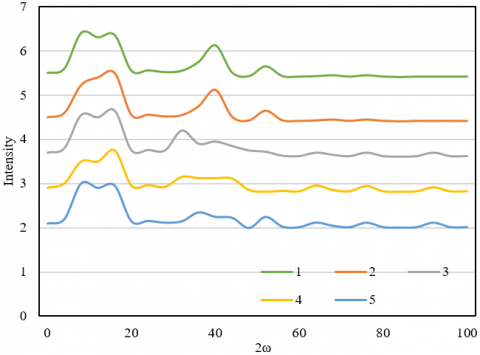(a)

## 5b.png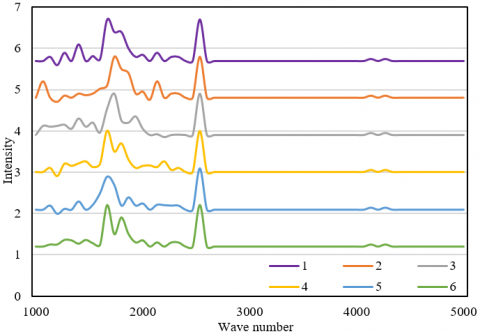(b)

Figure 5. XRD and ATR-FTIR images of different modified material samples

## 6.png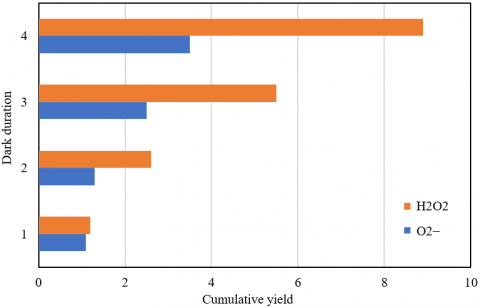Figure 6. Cumulative yield of final products H2O2 and $O_{2}^{-}$ under dark conditions

Table 5. The residual mass of the modified material with different mass losses at the temperature of 650℃

 Sample No polydopamine 3% 5% 7% Mass loss 6% 413.56 375.29 370.11 366.25 12% 400.23 362.54 356.42 362.15 650℃ (%) 0 2.14 4.74 5.59

Three common type strains, namely, Botrytis cinerea, Escherichia coli, and Staphylococcus aureus, were selected to verify the antibacterial activity of the prepared ZnO nanomaterial under dark conditions. Figure 6 shows the cumulative yield of final products H2O2 and $O_{2}^{-}$ under dark conditions. It can be observed that the prepared ZnO nanomaterial can suppress nearly 99.9% of the bacteria. The minimal inhibitory concentration was 200, 250, and 300 mg/L against the three common type strains. The performance is better than any ZnO nanostructure material available in the market. Table 5 shows the residual mass of the modified material with different mass losses at the temperature of 650℃. The results indicate that the pre-deposition of polydopamine improves the crystallinity of the modified material, and promotes the growth of ZnO nanocrystals. At 650℃, the residual mass of the modified material was 2.14g, 4.74g, and 5.59g, respectively, which is not very different from the actual dose.

The antibacterial activity of the prepared material, before and after the pre-deposition of polydopamine, against the three common type strains can be measured by the minimal inhibitory concentration. Figure 7 and Table 6 report the variation of minimal inhibitory concentration before and after pre-deposition of polydopamine. After the pre-deposition of polydopamine, the modified ZnO nanomaterial became stronger in antibacterial activity. Compared with the other two type strains, Staphylococcus aureus has a high resistance to modified ZnO nanomaterial. The main reason is that the carotenoid in Staphylococcus aureus enhances the resistance to ZnO oxidation stress to a certain extent.

To verify the safety of applying the prepared modified material in children’s furniture, this paper explores the toxicity of the material before and after pre-deposition of polydopamine to HUVECs by the CCK-8 method. The toxicity test results (Figure 8) show that the in vitro toxicity of the modified ZnO nanomaterial to HUVECs is dose-dependent. At a low concentration, the modified material promotes cell growth. The cytotoxicity of the modified ZnO nanomaterial hinges on the grain size, shape, and surface modification of its crystals. A high dose of the material greatly impacts the mitochondrial injury and apoptosis. Therefore, the modified ZnO nanomaterial should only be applied to children’s furniture in small quantities.

5. Conclusions

This paper discusses the preparation of a ZnO nanomaterial for children's furniture, and the research and development of antibacterial property. Based on the strengths and defects of ZnO nanomaterial, the authors specified the principle and flow of preparing Zinc nano-array for high-temperature hard materials, and wooden plates, respectively, and analyzed the antibacterial mechanism and antibacterial performance of the zinc nano-array. Then, the enrichment of ZnO nanocrystals on cellulose was improved by pre-depositing polydopamine particles. Through experiments, the particle size distribution of ZnO nanocrystals was analyzed; the particle size and antibacterial effect of ZnO crystals were determined at different concentrations of the growth solution; the XRD and ATR-FTIR images were plotted for different modified material samples; the cumulative yield of the final products, namely, H2O2 and $O_{2}^{-}$, were counted under dark conditions; the variation of minimal inhibitory concentration was plotted before and after pre-deposition of polydopamine. The experimental results show that the prepared modified material can achieve a good antibacterial performance.

Table 6. Minimal inhibitory concentrations before and after pre-deposition of polydopamine

 Strains Escherichia coli Staphylococcus aureus Botrytis cinerea Before After Before After Before After ZnO suspension concentration (mg/L) 10 + + + + + + 20 + - + + + + 30 + - + + + - 40 - + + + + - 50 - - + - + + 60 + + + + + + 70 + - - - + + 80 + - + + + - 90 + - + - - - 100 + - + + + - Positive reference + + + + + + Negative reference - - - - - -

## 7a.png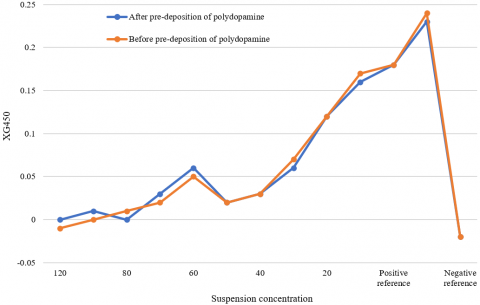## 7b.png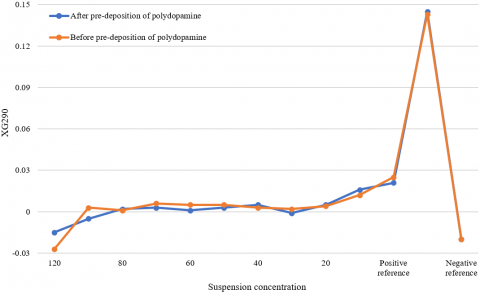Figure 7. The variation of minimal inhibitory concentration before and after pre-deposition of polydopamine

## 8.pngFigure 8. Toxicity test results of modified material on human umbilical vein endothelial cells (HUVECs)

References

 Christian, Y., Sahroni, T.R. (2020). Green productivity methodology for furniture industry. IOP Conference Series: Earth and Environmental Science, 426: 012159. https://doi.org/10.1088/1755-1315/426/1/012159

 Xu, X., Hua, Y., Wang, S., Xu, G. (2020). Determinants of consumer’s intention to purchase authentic green furniture. Resources, Conservation and Recycling, 156: 104721. https://doi.org/10.1016/j.resconrec.2020.104721

 Xu, X., Wang, S., Yu, Y. (2020). Consumer’s intention to purchase green furniture: Do health consciousness and environmental awareness matter? Science of the Total Environment, 704: 135275. https://doi.org/10.1016/j.scitotenv.2019.135275

 Zhang, X. (2013). Furniture design based on green ecological environment. In Informatics and Management Science II, pp. 283-289. https://doi.org/10.1007/978-1-4471-4811-1_38

 Scheithauer, U., Schwarzer, E., Tscharntke, F., Schmidt, T., Jegust, S., Richter, H.J., Moritz, T., Michaelis, A. (2015). New lightweight kiln furniture made by combination of ceramic green tapes and extrudates. Interceram-International Ceramic Review, 64(4): 204-208. https://doi.org/10.1007/BF03401124

 Dotson, S. (2015). Green furniture: An assesment of furniture society member work. Journal of Green Building, 10(3): 47-66. https://doi.org/10.3992/jgb.10.3.47

 Han, J., Li, J., Jiang, Y., Wang, L. (2021). Application of innovative technology in children furniture design. In E3S Web of Conferences, 236: 04059. https://doi.org/10.1051/e3sconf/202123604059

 Jiang, L., Cheung, V., Westland, S., Rhodes, P.A., Shen, L., Xu, L. (2020). The impact of color preference on adolescent children's choice of furniture. Color Research & Application, 45(4): 754-767. https://doi.org/10.1002/col.22507

 Dai, L., Xu, B. (2019). Research on the furniture design criteria for children’s psychological development in home environment. In International Conference on Human-Computer Interaction, Orlando, FL, USA, pp. 277-286. https://doi.org/10.1007/978-3-030-23538-3_21

 Wan, M., Zhang, Y., Ye, W. (2018). Consumer willingness-to-pay a price premium for eco-friendly children's furniture in Shanghai and Shenzhen, China. Forest Products Journal, 68(3): 317-327. https://doi.org/10.13073/FPJ-D-17-00050

 Deng, T., Sun, W., Zhang, R. (2018). Research on parent-child interaction system of intelligent children’s furniture based on application behavior analysis. International Conference on Intelligent Human Systems Integration, Dubai, United Arab Emirates, pp. 633-638. https://doi.org/10.1007/978-3-319-73888-8_98

 Ekaterinushkina, A.V., Zhdanova, N.S., Mishukovskaya, J.I. (2018). Study of functional features of furniture and equipment in the design of recreation of children’s polyclinics. IOP Conference Series: Materials Science and Engineering, 463(3): 032028. https://doi.org/10.1088/1757-899X/463/3/032028

 Andaç, T., Güzel, A. (2017). Attitudes of families with children towards eco-friendly designed furniture: Kayseri sample. BioResources, 12(3): 5942-5952. http://doi.org/10.15376/biores.12.3.5942-5952

 Lu, C.W., Lu, J.M. (2017). Evaluation of the Indonesian National Standard for elementary school furniture based on children's anthropometry. Applied Ergonomics, 62: 168-181. https://doi.org/10.1016/j.apergo.2017.03.004

 Dsingh, A. (2017). Degree of musculoskeletal pain and other discomforts experienced by school children using different type of furniture during class. International Conference on Research into Design, Guwahati, India, pp. 893-903. https://doi.org/10.1007/978-981-10-3518-0_77

 Wan, M., Toppinen, A. (2016). Effects of perceived product quality and lifestyles of health and sustainability (LOHAS) on consumer price preferences for children's furniture in China. Journal of Forest Economics, 22: 52-67. https://doi.org/10.1016/j.jfe.2015.12.004

 Yuan, Z.Y., Lei, Y.F., Dong, H., Meng, F.L. (2021) Sensitivity analysis of ZnO nanometer gas sensor based on dynamic temperature modulation. Journal of Northeastern University (Natural Science), 42(4): 469-477. https://doi.org/10.12068/j.issn.1005-3026.2021.04.003

 Hermida-Montero, L.A., Paraguay‑Delgado, F., Cruz, L. F., Carrillo, D., Mtz‑Enriquez, A.I., Pariona, N. (2021). The role of coating and size of ZnO nanoparticles on the antifungal activity against Raffaelea species. Materials Letters, 301: 130314. https://doi.org/10.1016/j.matlet.2021.130314

 La, D.D., Nguyen-Tri, P., Le, K.H., Nguyen, P.T., Nguyen, M.D.B., Vo, A.T., Nguyenh, M.T.H., Chang, S.W., Tran, L.D., Chung, W.J., Nguyen, D.D. (2021). Effects of antibacterial ZnO nanoparticles on the performance of a chitosan/gum Arabic edible coating for post-harvest banana preservation. Progress in Organic Coatings, 151: 106057. https://doi.org/10.1016/j.porgcoat.2020.106057

 Li, C., Wang, C., Li, Z., Cao, Z., Xie, Y., Xue, M., Zhao, J. (2021). Preparation of ZnO nanoparticle/acrylic resin superhydrophobic coating via blending method and its wear resistance and antibacterial properties. Materials, 14(14): 3775. https://doi.org/10.3390/ma14143775

 Tuong, V.M., Huyen, N.V., Kien, N.T., Dien, N.V. (2019). Durable epoxy@ ZnO coating for improvement of hydrophobicity and color stability of wood. Polymers, 11(9): 1388. https://doi.org/10.3390/polym11091388

 Pang, S., He, Y., Zhong, R., Guo, Z., He, P., Zhou, C., Xue, B., Wen, X., Li, H. (2019). Multifunctional ZnO/TiO2 nanoarray composite coating with antibacterial activity, cytocompatibility and piezoelectricity. Ceramics International, 45(10): 12663-12671. https://doi.org/10.1016/j.ceramint.2019.03.076